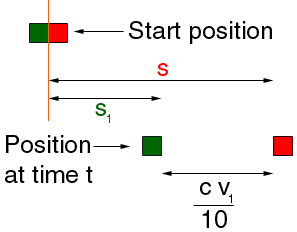Name: Lisa Who is asking: Student Question: A good rule of thumb when driving is that you should be about one car length away from the car in front of you for every 10 miles per hour that you are travelling. Suppose you follow this rule perfectly (so you are exactly the correct distance away). You are waiting at a stop light with your front bumper just touching the car in front of you. The light turns green and the car in front accelerates at a constant rate "r". Calculate how you should accelerate in order to follow the rule. Hi Lisa, Below is a diagram that shows the two cars, at time t = 0, just as the light changes, and at time t a short time later (your car is green, the other is red).The length of your car is c miles. At time t the red car is travelling v miles per hour, you are travelling at v1 miles per hour, the red car's acceleration is r miles per hour squared and your acceleration is r1 miles per hours squared . Also the red car has travelled s miles and you have travelled s1 miles. Since you must be "one car length away from the car in front of you for every 10 miles per hour", the distance from you to the red car is  c v1/10 miles. Hence, from the diagram s - s1 = c v1/10 Differentiating both sides with respect to t gives v - v1 = c r1/10 and differentiating again with respect to t gives r - r1 = c r'1/10 where r'1 is the dertvative of r1 with respect to t. Since r is a constant you can solve for r1. Penny Go to Math Central